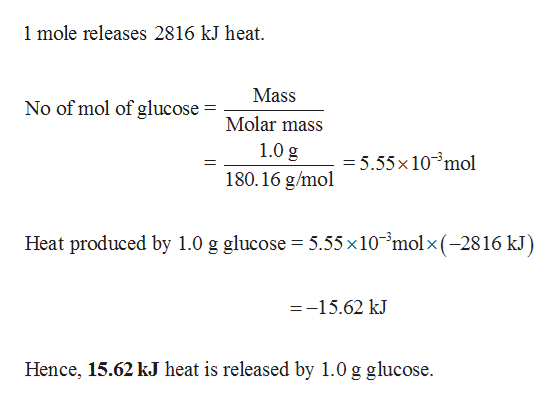# The oxidation of the sugar glucose, C6H12O6, is described by the following equation:C6 H12 O6(s) + 6O2(g) ⟶ 6CO2(g) + 6H2 O(l)    ΔH = −2816 kJThe metabolism of glucose gives the same products, although the glucose reacts with oxygen in a series of steps in the body.a) How much heat in kilojoules can be produced by the metabolism of 1.0 g of glucose?(b) How many Calories can be produced by the metabolism of 1.0 g of glucose?

Question
194 views

The oxidation of the sugar glucose, C6H12O6, is described by the following equation:

C6 H12 O6(s) + 6O2(g) ⟶ 6CO2(g) + 6H2 O(l)    ΔH = −2816 kJ
The metabolism of glucose gives the same products, although the glucose reacts with oxygen in a series of steps in the body.

a) How much heat in kilojoules can be produced by the metabolism of 1.0 g of glucose?

(b) How many Calories can be produced by the metabolism of 1.0 g of glucose?

check_circle

Step 1

(a)

Amount of heat (in kilojoules) produced by the metaboli...help_outlineImage Transcriptionclose1 mole releases 2816 kJ heat. Mass No of mol of glucose Molar mass 1.0 g 5.55x 10 mol 180.16 g/mol Heat produced by 1.0 g glucose 5.55x10 molx -2816 kJ) =-15.62 kJ Hence, 15.62 kJ heat is released by 1.0 g glucose fullscreen

### Want to see the full answer?

See Solution

#### Want to see this answer and more?

Solutions are written by subject experts who are available 24/7. Questions are typically answered within 1 hour.*

See Solution
*Response times may vary by subject and question.
Tagged in

### Chemical Thermodynamics